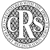The fountain of wisdomPublisher of books and software in mathematics and computer science
 Home Preface Report Register About us
Beginning Algebra

Intermediate Algebra

College Algebra

Pre-Calculus I
Pre-Calculus II
Pre-Calculus III

Calculus I
Calculus II
Calculus III

Functions, Differential and Integral Calculus
Trigonometry, Trigonometric functions and equations
Geometry and Use of Trigonometry
Analytic Geometry Conic Sections
Vectors in 2D and 3D
Coordinate Geometry  Points, Lines and Planes in 3D Space    Represented by Vectors
Matrices, Determinants, Systems of Linear Equations
Combinatorial Analysis and Probability

Solved Problems from the Beginning to High School
Solved Problems from High School to Master's Degree

Let's set up the missing cornerstone!
A revealing insight into the polynomial function
Every polynomial function has its initial position at the origin of the coordinate system
There are three methods to transform an n-th degree polynomial written in the general form,
f (x) = anxn + an - 1 xn - 1 + an - 2 xn - 2 + . . . + a2x2 + a1x + a0
to its source form
fs(x) = anxn + an - 2 xn - 2 + an - 3 xn - 3 + . . . + a3x3 + a2x2 + a1x,
whose graph passes through the origin.
Each method is based on the fact that a polynomial written in general form represents translation of its source (original) function in the direction of the coordinate axes, where the coordinates of translations areTherefore, each polynomial missing second term (an - 1 = 0), represents a source polynomial function whose graph is translated in the direction of the y-axis by  y0 = a0.
Let's reveal all three methods.
First method
If we plug the coordinates of translations x0 and y0 with changed signs into a given polynomial  y =  f (x), expressed in the general form, i.e.,
y + y0 = an(x + x0)n + an - 1(x + x0)n - 1 + . . . + a1(x + x0) + a0
then, after expanding and reducing the above expression we get its source function  fs(x).
Inversely, by plugging the coordinates of translations into the source polynomial function, i.e.,
y - y0 = an(x - x0)n + an - 2(x - x0)n - 2 + . . . + a2(x - x0)2 + a1(x - x0),
after expanding and reducing the above expression, we get given polynomial  f (x).
Note that the complete source polynomial has n - 1 terms, missing second and the absolute term.
Second method
The coefficients a, of the source polynomial function are related to corresponding value of the derivative of the given polynomial at x0, like coefficients of the Taylor polynomial in Taylor's or Maclaurin's formula, thuswhere,  an = anan - 1 = 0a0 =  f (x0), and  f (n - k)(x0) denotes the (n - k)-th derivative at x0.
Observe that coefficients of the source polynomial function define the value and the direction of the vertical translation of successive derivatives of given polynomial that is,
f (n - k)(x0) = (n - k)! an - k .
Further, the horizontal translation of each successive derivative corresponds with x0 of the given                 polynomial function.
For example, the coefficient a1 of the source cubic function  f (x) = a3x3 + a2x2 + a1x + a0
since   f ' (x) = 3a3x2 + 2a2x + a1   and   x0 = - a2/(3a3)  thenThus,              fs(x) = a3x3 + a1x   is the source cubic polynomial function,
where the coefficient a1 equals the slope of the tangent line at the inflection point I (x0, y0).
Further, as  a1 =  f ' (x0),  the coefficient a1 also represents the vertical translation of the first derivative of the cubic polynomial, that is why the sign of the product  a3a1 is used as the additional condition in the classification of the cubic polynomial shown later.New:  1. Trigonometry 1 Applications of Trigonometry:
-  the book is now available in paperback on the Amazon’s book store Please visit,:Registered with the IP Rights Office Copyright Registration Service Ref: 413180394